Here we are providing Class 12 Chemistry Important Extra Questions and Answers Chapter 3 Electrochemistry. Class 12 Chemistry Important Questions are the best resource for students which helps in Class 12 board exams.

## Class 12 Chemistry Chapter 3 Important Extra Questions Electrochemistry

### Electrochemistry Important Extra Questions Very Short Answer Type

Question 1.
Write the name of the cell which Is generally used in hearing aids. Write
the reactions taking place at the anode and the cathode of this cell. (CBSE AI 2017)
Mercury cell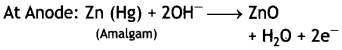At Cathode: HgO + H2O + 2e → Hg (I) + 2OH

Question 2.
Write the name of the cell which is generally used in transistors. Write the reactions taking place at the anode and the cathode of this cell. (CBSE Al 2017)
Dry cell
Anode: Zn (s) → Zn2+ + 2e
Cathode: MnO2 + NH4+ + e → MnO(OH) + NH3

Question 3.
Write the name of the cell which is generally used in inverters. Write the reactions taking place at the anode and the cathode of this cell. (CBSE Al 2017)
Anode: Pb (s) + SO42-(aq) → PbSO4(s) + 2e
Cathode: PbO2 + SO42-(aq) + 4H+ + 2e → PbSO4(s) + 2H2O(l)

Question 4.
From the given cells:
Lead storage cell, Mercury cell, Fuel cell and Dry cell Answer the following:
(i) Which cell is used in hearing aids?
(ii) Which cell was used in Apollo Space Programme?
(iii) Which cell is used in automobiles and inverters?
(iv) Which cell does not have long life? (CBSE Delhi 2016)
(i) Mercury cell
(ii) Fuel cell
(iv) Dry cell

Question 5.
What happens if external potential applied becomes greater than E°el, of electrochemical cell? (CBSE Al 2016)
If E°cell (external) is greater than E°cell, the cell starts acting as an electrolytic cell. In this case, electrical energy is used to carry out non-spontaneous chemical reaction.

Question 6.
Using the E° values of A and B, predict which one is better for coating the surface of iron [E°(Fe2+/Fe) = – 0.44V] to prevent corrosion and why?
Given: E°(A2+|A) = -2.37 V and E°(B2+|B) = – 0.14 V (CBSE Al 2016)
‘A’ will prevent iron from rusting. So, we can coat the iron surface with metal A because it has more negative value.

How to calculate emf of a cell Calculate the emf of the cell in which the following reaction takes place.

Question 7.
Given that the standard electrode potentials (E°) of metals are:
K+/K = – 2.93V, Ag+/Ag = 0.80V, Cu2+/Cu = 0.34V, Mg2+/Mg = – 2.37V, Cr3+/Cr = – 0.74V and Fe2+/Fe = – 0.44V. (CBSE Al 2010)
Arrange the metals in the increasing order of their reducing power.
Ag+/Ag < Cu2+/Cu < Fe2+/Fe < Cr3+/Cr < Mg2+/Mg < K+/K.

Question 8.
What is change in free energy for
(a) galvanic cell and
(b) electrolytic cell?
(a) For a galvanic cell, free energy decreases, i.e. ΔG < 0.
(b) For electrolytic cell, free energy increases, i.e. ΔG > 0.

Question 9.
What is role of ZnCl2 in a dry cell?
ZnCl2 combines with NH3 produced to form the complex [Zn(NH3)2Cl2], otherwise the pressure developed due to NH3 would crack the seal of the cell.

Question 10.
When the silver electrode having reduction potential 0.80 V is attached to NHE, will it act as anode or cathode?
It will act as cathode.

Question 11.
What is the effect of carbon dioxide in water on corrosion?
The presence of carbon dioxide in water increases rusting of iron. Water containing CO2 acts as an electrolyte and increases the flow of electrons from one place to another.

Question 12.
Why is it not possible to measure the voltage of an isolated half reaction?
It is not possible to measure the voltage of an isolated half reaction because neither the oxidation nor the reduction can occur by itself. Therefore, we can only calculate the relative electrode potential by connecting it to some standard electrode.

Question 13.
Why does a dry cell become dead after a long time, even if it has not been used?
A dry cell becomes dead after a long time because the acidic NH4Cl corrodes the zinc container.

Question 14.
Why does the cell potential of mercury cell remain constant throughout its life? (CBSE AI 2015)
This is because the overall cell reaction does not involve any ion in the solution whose concentration changes during its life time.

Question 15.
How can you increase the reduction potential of an electrode?
By increasing the concentration of the ions

Question 16.
The E° values of MnO4, Ce4+ and Cl2 are 1.507, 1.61 and 1.358 V respectively. Arrange these in order of increasing strength as oxidising agent.
Cl2 < MnO4 < Ce4+.

Question 17.
E° values for Fe3+/Fe2+ and Ag+/Ag are respectively 0.771 V and 0.800 V. Is the reaction:
Fe3+ + Ag → Fe2+ + Ag+ spontaneous or not?
E° for the reaction is 0.771 – 0.800 = – 0.029 V.
Therefore, the reaction is not spontaneous.

Question 18.
What is the use of platinum foil in the hydrogen electrode?
It is used for inflow and outflow of electrons.

Question 19.
What is meant by limiting molar conductivity? (CBSE 2010)
The molar conductivity at infinite dilution or when concentration approaches zero is called limiting molar conductivity.

Question 20.
Express the relation among the conductivity of solution in the cell, the cell constant and the resistance of solution in the cell. (CBSE Delhi 2011)
The conductivity (K), cell constant (G°) and resistance (R) of the solution are related as:
K = G° × 1/R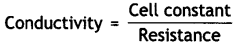Question 21.
Explain the relation between conductivity and molar conductivity of a solution held in a ceil. (CBSE Delhi 2011)
Conductivity (κ) and molar conductivity are related as:
m = $$\frac{\kappa \times 1000}{M}$$
where M is the molarity of the solution.

Question 22.
Is it safe to stir 1 M AgNO2 solution with a copper spoon?
Given E° (Ag+/Ag) = 0.80 V, E° (Cu2+/Cu) = 0.34 V. Explain.
No, copper spoon will dissolve as Cu2+ ions because copper has more tendency to get oxidised than silver.

Question 23.
The e.m.f. of the cell:
Zn | Zn2+ (1M) || H+ (1M) | H2 (1 atm),
Pt is 0.76 V. What is the electrode potential of Zn2+/Zn electrode?
cell = E°(H+/H2) – E°(Zn2+/Zn)
0. 76 = 0 – E°(Zn2+/Zn)
∴ E°(Zn2+/Zn) = – 0.76 V.

Question 24.
Write relationship between
(i) standard free energy change and e.m.f. of a cell.
(ii) standard free energy change and equilibrium constant.
(i) ∆G° = – nFE°cell
(ii) ∆G° = – RT In Kc

Question 25.
Give the units of specific conductance and molar conductance.
Specific conductance: ohm-1 cm-1
Molar conductance: ohm-1 cm2 mol-1

Question 26.
Give one example each of primary cell and secondary cell.
Primary cell: Dry cell

Question 27.
How are cell constant and specific conductance related to one another?
Specific conductance = Cell constant × Conductance.

Question 28.
Why is the equilibrium constant K related to only E°cell and not Ecell?
This is because at equilibrium Ecell = 0.

Question 29.
What is the effect of decreasing concentration on the molar conductivity of weak electrolytes?
With the decrease in concentration of weak electrolytes, the molar conductivity increases.

Question 30.
Why is it not possible to determine the potential of a single electrode?
Oxidation and reduction cannot occur alone. Therefore, it is not possible to measure single electrode. Moreover, it is a relative tendency and can be measured with respect to a reference electrode only.

Question 31.
Suggest a metal which can be used for cathodic protection of iron?
Zinc.

Question 32.
Write the overall cell reaction for lead storage battery.
The overall cell reaction is:
Pb (s) + PbO2 (s) + 4H+ (aq) + 2SO42- (aq) → 2PbSO4 (s) + 2H2O.

### Electrochemistry Important Extra Questions Short Answer Type

Question 1.
The resistance of a conductivity cell containing 0.001 M KCI solution at 298 K is 1500 Ω. What is the cell constant if the conductivity of 0.001 M KCl solution at 298 K is 0.146 × 10-3 S cm-1? (CBSE AI 2008; CBSE Delhi 2007, 2008, 2012)
Ans.
Conductivity, K = 0.146 × 10-3 S cm-1
Resistance, R = 1500 ohm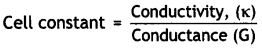= Conductivity (κ) × Resistance (R)
∴ Cell constant = 0.146 × 10-3 ohm-1 cm-1 × 1500 ohm = 0.219 cm-1.

Question 2.
The conductivity of 0.20 M KCl solution at 298 K is 0.025 S cm-1. Calculate its molar conductivity.
Molar conductivity
m = $$\frac{\kappa \times 1000}{\mathrm{C}}$$
k = 0.025 cm-1, C = 0.20 M
∴ ∧m = $$\frac{0.025 \times 1000}{0.20}$$ = 125.0 S cm2 mol-1

Question 3.
Resistance of a conductivity cell filled wIth 0.1 M KCl solution is 100. If the resistance of the same cell when filled with 0.02 M KCl solution is 520Ω, calculate the conductivity and molar conductivity of 0.02 M KCl solution. (The conductivity of 0.1 M KCl solution is 1.29 S m-1.) (CBSE AI 2006, CBSE Delhi 2014)
Step 1. Let us first calculate the cell constant.
Cell constant, G* = Conductivity (κ) × Resistance (R)
Resistance of 0.1 M KCl solution = 100 Ω
Conductivity of 0.1 M KCl solution = 1.29 S m-1
∴ Cell constant = 1.29 (S m-1) × 100Ω = 129 m-1
or = 1.29 cm-1

Step II. Calculation of conductivity of 0.02 M KCl soLution.
Resistance of solution = 520 Ω
Cell constant (G*) = 1.29 cm-1Step III. Calculation of molar conductivity.
m = $$\frac{1000 \times \kappa}{C}$$
C = 0.02 M, κ = 0.248 × 10-2 S cm-1
∴ ∧m = $$\frac{1000 \times 0.248 \times 10^{-2}}{0.02}$$ – 124 S cm2 mol-1

Question 4.
The electrical resistance of a column of 0.05 M NaOH+ solution of diameter 1 cm and length 50 cm is 5.55 × 103 ohm. Calculate its
(i) resistivity
(ii) conductivity, and
(iii) molar conductivity. (CBSE AI 2012)
Cell constant, G* = $$\frac{1}{a}$$
l = 50 cm, diameter = 1 cm
Area of cross-section, a = πr2
= 3.14 × (0.5)2 = 0.785 cm2
∴ G* = $$\frac{50}{0.785}$$ = 63.694 cm-1

(i) Resistivity,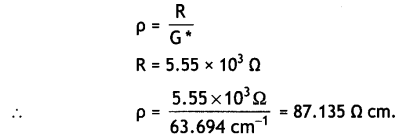(ii) Conductivity,
κ = $$\frac{1}{\rho}=\frac{1}{87.135}$$ = 1.48 × 10-2 cm-1

(iii) Molar conductivity,
m = $$\frac{\kappa \times 1000}{\mathrm{C}}$$
c = 0.05 M
∴ ∧m = $$\frac{1.148 \times 10^{-2} \times 1000}{0.05}$$ = 229.6 s cm2 mol-1

Question 5.
The molar conductivities at infinite dilution for sodium acetate, hydrochloric acid and sodium chloride are 91.0, 425.9 and 126.4 S cm2 mol-1 respectively at 298 K. Calculate the molar conductivity of acetic acid at infinite dilution. (CBSE Delhi 2010)
Molar conductivity at infinite dilution for acetic acid can be calculated as:
∧° (CH3COOH ) = λ°H+ + ∧°CH3COO
= λCH3COO + λ°Na+ + λ°H+ + λ°cl – λ°Na+ – λ°cl
∧°(CH3COOH+) = ∧°(CH3COONa) + ∧° (H+Cl) – ∧° (NaCl)
∧°(CH3COONa) = 91.0 S cm2 mol-1
∧°(H+Cl) = 425.9 S cm2 mol-1
∧°(NaCl) = 126.4 S cm2 mol-1
∴ ∧°(CH3COOH+) = 91.0 + 425.9 – 126.4 = 390.5 S cm2 mol-1.

Question 6.
(a) Write the reaction that occurs at anode on electrolysis of concentrated H2SO4 using platinum electrodes.
(b) What is the effect of temperature on ionic conductance? (CBSE Al 2019)
(a) At anode:
2SO42-(aq) → S2O82-(aq) + 2e
(b) Ionic conductance will increase with increase in temperature.

Question 7.
Write anode and cathode reactions that occur in dry cell. How does a dry cell differ from a mercury cell? (CBSE Al 2019)
Anode: Zn → Zn2+ + 2e
Cathode:
NH4+(aq) + MnO2(s) + e → MnO(OH) + NH3
The potential of a dry cell decreases slowly but continuously, while the potential of mercury cell remains constant throughout its life.

Question 8.
Calculate the limiting molar conductivity of CaS04 if limiting molar conductivities of calcium and sulphate ions are 119.0 and 106.0 S cm2 mol-1 respectively. (CBSE Sample Paper 2012)
∧°m (CaSO4) = λm (Ca2+) + λ°m (SO42-)
= 119.0 + 106.0
= 225.0 S cm2- mol-1.

Question 9.
Arrange the following solutions in the decreasing order of specific conductance.
(i) 0.01M NaCl (ii) 0.05M NaCl (iii) 0.1M NaCl (iv) 0.5M NaCl
Ans.
(iv) 0.5M NaCl > (iii) 0.1M NaCl > (ii) 0.05M NaCl > (i) 0.01M NaCl

Question 10.
Write the Nernst equation and calculate the e.m.f. of the following cell at 298 K:
Cu(s) | Cu2+ (0.130 M) | | Ag+ (1.0 × 10-4 M)) | Ag (s)
Given: E(Cu2+/Cu) = + 0.34V and E(Ag2+/Ag) = + 0.80V (CBSE Al 2004)
The electrode reactions and cell reaction are:Question 11.
Calculate Ecell, for the following reaction at 298K:
2Cr(s) + 3Fe2+ (0.01 M) → 2Cr3+ (0.01M) + 3Fe(s)
(Given: Ecell = 0.261v)                (CBSE AI 2016)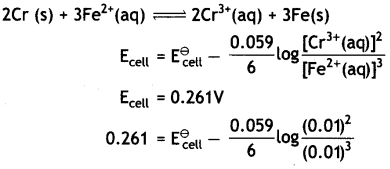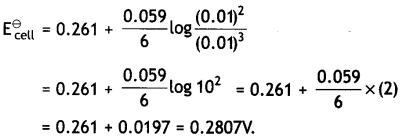Question 12.
For the reaction:
2AgCl (s) + H2 (g) (1atm) → 2Ag(s) + 2H+ (0.1M) + 2Cl (0.1M)
ΔG° = – 43600 J at 25°C
Calculate the e.m.f of the cell. (CBSE AI 2018)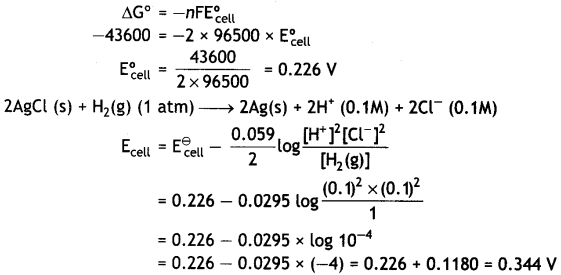Question 13.
Write the name of two fuels other than hydrogen used in fuel cell. Write two advantages of fuel cell over an ordinary cell. (CBSE Al 2019)
Methane, oxygen
(i) Fuel cells have greater efficiency than ordinary cells.
(ii) These do not produce any harmful by-product and therefore, do not cause any pollution.

Question 14.
Define conductivity and molar conductivity for the solution of an electrolyte. Why does the conductivity of solution decrease with dilution? (CBSE 2019C)
Conductivity of a solution at any given concentration is the conductance of one unit volume of solution kept between two platinum electrodes with unit area of cross section and at a distance of unit length.

Molar conductivity of a solution at a given concentration is the conductance of the volume V of solution containing one mole of electrolyte kept between two electrodes with area of cross section A and distance of unit length. Therefore,
m = $$\frac{\mathrm{κA}}{\mathrm{l}}$$
Since l = 1 and A = V (Volume containing 1 mole of electrolyte).
m = κV
Conductivity always decreases with decrease in concentration both for weak and strong electrolytes. It is because the number of ions per unit volume that carry the current in solution decreases on dilution.

Question 15.
Express the relation among cell constant, resistance of the solution in the cell and conductivity of the solution. How is molar conductivity of a solution related to its conductivity? (CBSE AI 2012)
The conductivity (κ), cell constant (G*) and resistance (R) of solution are related as: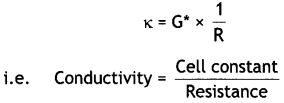Molar conductivity (∧m) is related to conductivity (κ) as:
m = $$\frac{\kappa \times 1000}{M}$$

Question 16.
The molar conductivity of a 1.5 M solution of an electrolyte is found to be 138.9 S cm2 mol-1. Calculate the conductivity of this solution. (CBSE AI 2012)
m = 138.9 S cm2 mol-1, M = 1.5 M
138.9 = $$\frac{\kappa \times 1000}{1.5}$$
∴ κ = $$\frac{138.9 \times 1.5}{1000}$$ = 0.208 ohm-1 cm-1.

### Electrochemistry Important Extra Questions Long Answer Type

Question 1.
(a) Following reaction takes place in the cell:
Zn (s) + Ag2O (s) + H2O (l) → Zn2+ (aq) + 2Ag (s) + 20H (aq)
Calculate ΔrG° of the reaction.
[Given: E°(Zn2+/Zn) = – 0.76 V,
(Ag2+/Ag) = 0.80 V, 1 F = 96,500 C mol-1]
cell = E°(Ag2+/Ag) – E°(Zn2+/Zn)
= 0.80 – (-0.76) = 1.56V
ΔG° = -nFE°cell          (Here n = 2)
= – 2 × 96500 × 1.56
= – 301080 J mol-1
or = – 301.080 kJ mol-1

(b) How can you determine limiting molar conductivity (∧m°,) for strong electrolyte and weak electrolyte? (CBSE Al 2019)
For strong electrolytes ∧m° can be obtained as intercept from the plot of Am vs C-1/2 graph. For weak electrolytes ∧m° can be obtained from Kohlrausch law.

Question 2.
(i) Explain the following:
(a) CO2 is always present in natural water. Explain its effect (increases, stops or no effect) on rusting of iron.
(b) Rusting of iron is quicker in saline water than in ordinary water. Explain.
(i) (a) Presence of CO2 in natural water increases rusting of iron. It dissolves in water to form H2CO3 which gives H+ ions. The H+ ions accelerate the process of corrosion.
In rusting of iron, Fe oxidises to Fe2+ ions
Fe (s) → Fe2+ (aq) + 2e (anode)
The released electrons go to the cathode and reduce oxygen in the presence of H+ ions (obtained from H2CO3). The reaction occurs at cathode. Thus, CO2 increases rusting.
O2 (g) + 4H+(aq) + 4e → 2H2O (l)

(b) Rusting of iron is quicker in saline water (salt water) than in ordinary water. This is mainly due to the fact that saline water increases the electrical conduction of electrolyte solution formed on the metal surface. Therefore, rusting becomes more serious problem where salt water is present.

(ii) Discuss electrical protection for preventing rusting of iron pipes in underground water.
In this method iron articles which are in contact with water such as underground water pipes are protected from rusting. The article of iron is connected with more active metals like magnesium or zinc. This prevents its tendency to lose electrons and therefore, corrosion is prevented. The cathodes of magnesium of zinc can be fixed to the surface of iron or burned in sub-soil water near by the pipes.

Question 3.
Give the construction and working of hydrogen standard electrode potential?
The standard hydrogen electrode consists of platinum wire sealed in a glass tube and has a platinum foil attached to it. The foil is coated with finely divided platinum and acts as platinum electrode. It is dipped into an acid solution containing H+ ions in 1 M concentration (1 M HCl). Pure hydrogen gas at 1 atmosphere pressure is constantly bubbled into the solution at constant temperature of 298 K. The surface of the foil acts as a site for the reaction. This is shown in figure.
The following reactions occur in this half cell depending upon whether it acts as an anode or as a cathode: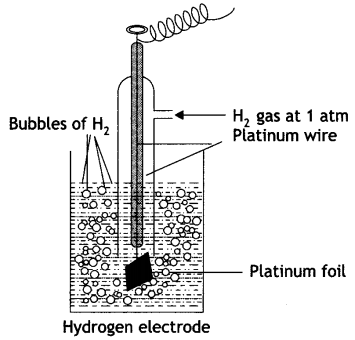If S.H.E. acts as anode:
H2(g) → 2H+ + 2e
If S.H.E. acts as cathode:
2H+ + 2e → H2(g)
The electrode potential of an electrode can be determined by connecting this half cell with a standard hydrogen electrode. The electrode potential of the standard hydrogen electrode is taken as zero.

Measurement of the standard electrode potential (E°): The standard electrode potential of a metal electrode is measured with respect to a standard hydrogen electrode. A cell is prepared in which the metal electrode constitutes one half cell and the S.H.E. as the other half cell (anode). The electrons released by the metal in the oxidation half cell are accepted by the H+ ions of the acid in the reduction half cell.
The cell may be represented as:
Pt(s) | H2(g, 1 atm) | H+ (aq, 1 M) || Mn+ (aq, 1M) | M
Now, e.m.f. of cell
e.m.f. = ER – EL
Since the potential of S.H.E. has been fixed to be zero, i.e. EL = 0 so that
e.m.f. = ER – 0
or ER = e.m.f.
From the knowledge of e.m.f. of the cell, the electrode potential of the electrode can be calculated. For example, if we wish to determine the electrode potential of zinc electrode in 1 M solution of ZnSO4, it is combined with S.H.E. The e.m.f. of the cell is found to be – 0.76 V so that
e.m.f. = EL – EL
– 0.76 = ER – 0
or ER = – 0.76 V.

Question 4.
What are fuel cells? Discuss briefly hydrogen-oxygen fuel cell?
Fuel cells. These are voltaic cells in which the reactants are fed continuously to the electrodes. These are designed to convert the energy from the combustion of fuel such as H2, CO, CH4, etc. directly into electrical energy. The common example is hydrogen-oxygen fuel cell as described below:Fig. A simple H2 – O2 fuel cell.

In this cell, hydrogen and oxygen are bubbled through a porous carbon electrode into concentrated aqueous sodium hydroxide as shown in figure. The diffusion rates of the gases into the cell are carefully regulated to get maximum efficiency. In the anode compartment hydrogen is oxidised while oxygen in the cathode compartment is reduced. The net reaction is the same as burning of hydrogen and oxygen to form water.
The reactions are given below:
At anode:
2 [H2 (g) + 2OH(aq) → 2H2O (l) + 2e]

At cathode:
O2 (g) + 2H2O (l) + 4e → 4OH(aq)

Overall reaction:
2H2 (g) + O2(g) → 2H2O (l)
The catalysts (Pt, Ag or Co) are also added.
This cell runs continuously as long as the reactants are fed. These fuel cells are more efficient than conventional methods of generating electricity on a large scale by burning hydrogen, carbon fuels because these fuel cells convert the energy of the fuel directly into electricity.
The cell has been used for electric power in the Apollo space programme.

Question 5.
What is corrosion? What are the factors which affect corrosion?
Corrosion. It is a process of eating away of metals when exposed to the atmosphere surrounding it. Many metals when exposed to the atmosphere, react with air or water in the environment to form undesirable compound on their surface. In case of iron, the corrosion is called rusting. The red or orange coating that forms on the surface of iron when exposed to air and moisture is called rust. Chemically, rust is a hydrated form of ferric oxide, Fe2O3 . xH2O.
Factors which affect corrosion. The main factors which affect corrosion are:
1. Position of metals in e.m.f. series: The reactivity of a metal depends upon its position in the electrochemical series. More the reactivity of the metal, more will be the possibility of the metal getting corroded.

2. Presence of impurities in metals: The impurities help in setting up a voltaic cell, which increases the speed of corrosion.

3. Presence of electrolytes: Presence of electrolytes in water also increases the rate of corrosion. For example, corrosion of iron in sea water takes place to larger extent than in distilled water.

4. Presence of CO2 in water. Presence of CO2 in natural water increases rusting of iron. Water containing CO2 acts as an electrolyte and increases the flow of electrons from one place to another.

5. Presence of protective coatings. When the iron surface is coated with layers of metals more active than iron, then the rate of corrosion is retarded. For example, coating of zinc on iron prevents rustings.

Question 6.
What type of battery is lead storage battery? Write the anode and the cathode reactions and the overall reaction occurring in a lead storage battery. (CBSE 2011)
It is a secondary cell.
Anode reaction:
Pb (s) + SO42- (aq) → PbSO4 (s) + 2e

Cathode reaction:
PbO2 (s) + 4H+ (aq) + SO42- (aq) + 2e → PbSO4 (s) + 2H2O (l)

Overall reaction:
Pb (s) + PbO2 (s) + 2H2S04 → 2PbSO4 (s) + 2H2O (l)

Question 7.
The chemistry of corrosion of iron is essentially an electrochemical phenomenon. Explain the reactions occurring during the corrosion of iron in the atmosphere. (CBSE 2011)
The chemistry of corrosion of iron is an electrochemical theory which involves oxidation and reduction reactions. According to this theory it is believed that non-uniform surface of metal or impurities present in iron behave like small electric cells (called corrosion couples) in the presence of water containing dissolved oxygen or carbon dioxide. A film of moisture with dissolved CO2 acts as electrolytic solution covering the metal surface at various places. This is shown in Fig. In these small electrolytic cells, pure iron acts as anode while cathodes are impure portions. The overall rusting involves the following steps:
Oxidation occurs at the anode of each electrochemical cell. Therefore, at each anode iron is oxidised to Fe2+ ions.

At anode:
Fe (s) → Fe2+ (aq) + 2e …… (i)
Thus, the metal atoms in the lattice pass into the solution as ions, leaving electrons on the metal itself. These electrons move towards the cathode region through the metal.

At the cathode of each cell, the electrons are taken up by hydrogen ions (reduction takes place). The H+ ions are obtained either from water or from acidic substance in water:
H2O ⇌ H+ + OH …… (ii)
or CO2 + H2O → H+ + H+CO3 …… (iii)

At cathode:
H+ + e → H+ …… (iV)
Thus, hydrogen atoms on the iron surface reduce dissolved oxygen.
4H + O2 → 2H2O ……..(v)
Therefore, the overall reaction at cathode of different electrochemical cells may be written as:
4H+(aq) + O2 (g) + 4e → 2H2O (l) ……. (vi)
The overall redox reaction may be written by multiplying reaction at anode Eq. (i) by 2 and adding reaction at cathode Eq. (iv) to equalise number of electrons lost and gained, i.e. Oxidation half reaction:
Fe (s) → Fe2+ (aq) + 2e × 2

Reduction half reaction:
4H+(aq) + O2 (g) + 4e → 2H2O (l)

Overall cell reaction:
2Fe (s) + 4H+(aq) + O2 (g) → 2Fe2+(aq) + 2H2O (l)
The ferrous ions are oxidised further by atmospheric oxygen to Fe3+ (as Fe2O3) and form rust
4Fe2+ + O2 (g) + 4H2O → 2Fe2O3 + 8H+
and Fe2O3 + xH2O → Fe2O3. xH2O
The H+ ions produced above are also used for reaction (iv).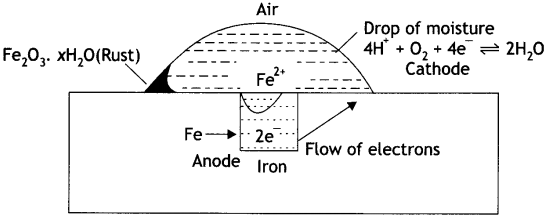Mechanism of rusting of air.

Question 8.
State Kohlrausch law of independent migration of ions. Why does the conductivity of a solution decrease with dilution? (CBSE 2014)
Kohlrausch law of independent migration of ions states that at infinite dilution when the dissociation is complete, each ion makes a definite contribution towards molar conductivity of the electrolyte irrespective of the nature of the other ion with which it is associated. Kohlrausch law also means that the limiting molar conductivity of an electrolyte is sum of the individual contributions of the ions of the electrolyte.

Conductivity (κ) of the electrolyte solution decreases with dilution because the number of ions per unit volume furnished by an electrolyte decreases with dilution.

Question 9.
(i) Following reactions occur at cathode during the electrolysis of aqueous silver chloride
solution:
Ag+(aq) + e → Ag(s)        E° = + 0.80 V
H+(aq) + e → $$\frac{1}{2}$$H2(g)         E ° = 0.00 V
On the basis of their standard electrode potential (E°) values which reaction is feasible at the cathode and why?
(ii) Define limiting molar conductivity. Why does conductivity of an electrolyte solution decrease with the decrease in concentration? (CBSE Delhi 2015)
(i) The reaction, Ag+(aq) + e → Ag(s) (E° = + 0.80 V) is feasible at cathode because its reduction potential is higher than other reaction (H+ + e → $$\frac{1}{2}$$ H2; E° = 0.0 V)

(ii) The molar conductivity of the solution when the concentration approaches zero (infinite dilution) is called limiting molar conductivity. Conductivity is the conductance of one centimeter cube of the solution. Upon diluting the solution, the concentration of ions per centimeter cube decreases and hence conductivity decreases with dilution.

Question 10.
(i) Following reactions occur at cathode during the electrolysis of aqueous sodium chloride
solution:
Na+(aq) + e → Na(s)        E° = -2.71V
H+(aq) + e → H2(g)         E ° = 0.00 V
On the basis of their standard reduction electrode potential (E°) values, which reaction is feasible at the cathode and why?
(i) The reaction: H(aq) + e → H2(g) (E° = 0.00 V) is feasible at cathode because its reduction
potential is higher than the other electrode reaction.

(ii) Why does the cell potential of mercury cell remain constant throughout its life? (CBSE 2015)
The cell potential of mercury cell remains constant because the overall reaction does not involve any ion in the solution whose concentration changes during its life time.

Question 11.
Zinc rod is dipped in 0.01 M solution of zinc sulphate when temperature is 298 K. Calculate the electrode potential of zinc.
(Given: E°zn2+/Zn = – 0.76 V; log 10 = 1) (CBSE 2019 C)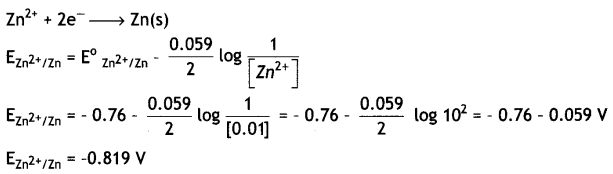Question 12.
Write Nernst equation and calculate e.m.f. of the following cells at 298 K:
(i) Mg (s) | Mg2+ (0.001 M) | | Cu2+ (0.0001 M) | Cu (s)
Given: EMg2+/Mg = -2.37 V, ECu2+/Cu = 0.34V
(i) The electrode reactions and cell reactions are: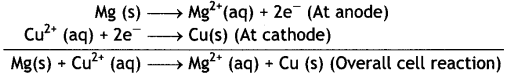Since the reaction involves 2 moles of electrons and therefore, n = 2 and the Nernst equation for the cell at 298 K is: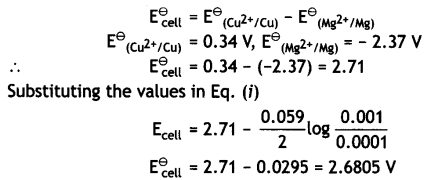(ii) Fe (s) | Fe2+ (0.001 M) | | H+ (1M) | H2 (1 atm) | Pt
Given: EFe2+/Fe = -0.44V (CBSE Delhi 2013)
Fes | Fe2+ (0.001 M) || H+ (1 M) | H2 (1 atm) | Pt
The electrode reactions and overall cell reactions are: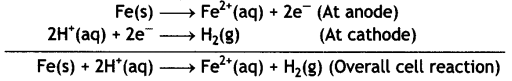Since the reaction involves 2 moles of electrons, therefore, n = 2 and the Nernst equation at 298 K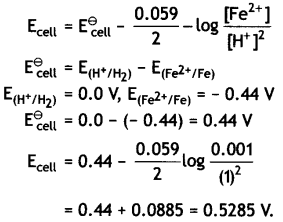(iii) Sn (s) | Sn2+ (0.050 M) | | H+ (0.020 M) | H2 (1 atm) | Pt
Given: ESn2+/Cn = – 0.14V (CBSE Al 2018)
The cell is:
Sn(s) | Sn2+(0.050M) | | H+(0.020 M) | H2(1 atm) | Pt
The electrode reactions and cell reactions are:The reaction involves 2 moles of electrons, therefore, n = 2 and the Nernst equation is: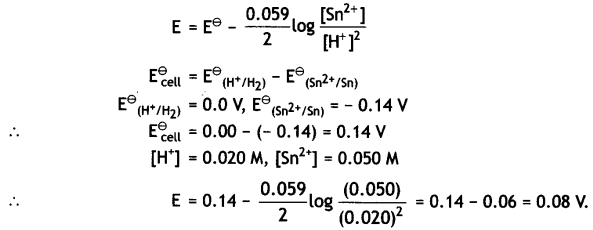Question 13.
(a) The e.m.f. of the following cell at 298 K is 0.1745 V:
Fe (s) | Fe2+ (0.1 M) | | H+ (x M) | H2 (g) (1 bar) | Pt (s)
Given: E°Fe2+Fe = – 0.44 V
Calculate the H+ ions concentration of the solution at the electrode where hydrogen is being produced.
(a)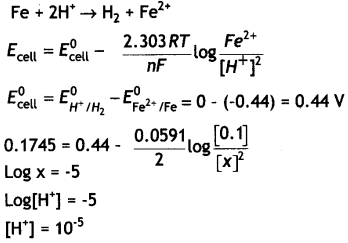(b) Aqueous solutions of copper sulphate and silver nitrate are electrolysed by 1 ampere current for 10 minutes in separate electrolytic cells. Will the mass of copper and silver deposited on the cathode be same or different? Explain your answer.
The mass of copper and silver deposited at the cathode will be different.
Faraday’s second law of electrolysis: It states that when same quantity of electricity is passed through different electrolytic solutions connected in series, the weights of the substances produced at the electrodes are directly proportional to their chemical equivalent weights.

For example, when same current is passed through two electrolytic solutions, containing copper sulphate (CuSO4) and silver nitrate (AgNO3) connected in series, the weights of copper and silver deposited are: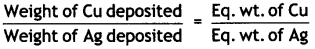OR

(a) Calculate the degree of dissociation of 0.0024 M acetic acid if conductivity of this solution is 8.0 × 10-5 S cm-1.
Given λ°H+ = 349.6 S cm2 mol-1; λ°CH3COO = 40.9 S 2 mol-1
(a)Electrolyte B is a strong electrolyte.

(b) Solutions of two electrolytes ‘A’ and ‘B’ are diluted. The limiting molar conductivity of ‘B’ increases to a smaller extent while that of ‘A’ increases to a much larger extent comparatively. Which of the two is a strong electrolyte? Justify your answer. (CBSE Sample Paper 2019)
Limiting molar conductivity increases only to a smaller extent for a strong electrolyte, as on dilution the interionic interactions are overcome. Limiting molar conductivity increases to a larger extent for a weak electrolyte, as on dilution the degree of dissociation increases, therefore the number of ions in total volume of solution increases. Therefore, ‘B’ is a strong electrolyte.

Question 14.
(i) Calculate E°cell for the following reaction at 298 K:
2Cr(s) + 3Fe2+ (0.01 M) → 2Cr3+ (0.01 M) + 3Fe(s)
Given: Ecell = 0.261 V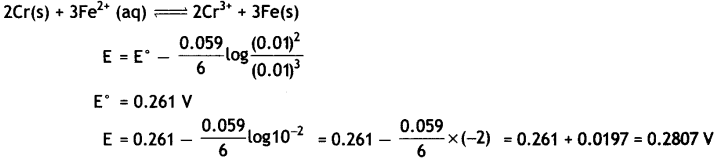(ii) Using the E° values of A and B, predict which one is better for coating the surface of iron [Ee°Fe2+/Fe) = – 0.44 V] to prevent corrosion and why?
Given: E°(A2+/A) = -2.37 V: E°(B2+/B) = – 0.14 V
‘A’ will prevent iron from corrosion. So, we can coat the iron surface with metal A because it has more negative E° value.

OR

(i) The conductivity of 0.001 mol L-1 solution of CH3COOH Is 3.905 × 10-5 S cm-1. Calculate its molar conductivity and degree of dissociation (α).
Given λ°(H+) = 349.65 cm2 mol-1 and λ°(CH3COO) = 40.9 S cm2 mol-1.(ii) Define electrochemical cell. What happens if external potential applied becomes greater than E°cell of electrochemical cell? (CBSE 2016)
Electrochemical cell is a device used for the production of electricity from energy released during spontaneous chemical reaction. Electrochemical cell converts chemical energy into electrical energy.
If E°cell (external) > E°cell, the cell starts acting as an electrolytic cell. In this case, electrical energy is used to carry out non-spontaneous chemical reaction.

Question 15.
(i) Define the following terms:
(a) Limiting molar conductivity
(b) Fuel cell
(ii) Resistance of a conductivity cell filled with 0.1 mol L-1 KCl solution is 100 Ω. If the resistance of the same cell when filled with 0.02 mol L-1 KCl solution is 520 Ω, calculate the conductivity and molar conductivity of 0.02 mol L-1 KCl solution. The conductivity of 0.1 mol L-1 KCl solution is 1.29 × 10-2-1 cm-1.

OR

(i) State Faraday’s first law of electrolysis. How much charge in terms of Faraday is required for the reduction of 1 mol of Cu2+ to Cu.
(ii) Calculate emf of the following cell at 298 K:
Mg(s) | Mg2+(0.1 M) | | Cu2+(0.01)|Cu(s) [Given E°cell = +2.71 V, 1 F = 96500 C mol-1] (CBSE Delhi 2014)
(a) The molar conductivity of a solution when concentration approaches zero is called limiting molar conductivity and is expressed as ∧°m.

(b) Fuel cells are voltaic cells in which the reactants are continously supplied to the electrodes and are designed to convert energy from the combustion of fuels such as H2, CO, CH4, etc. directly into electrical energy.

(ii) Let us first calculate cell constant
Cell constant, G* = Conductivity (κ) × Resistance (R)
Resistance of 0.1 M KCl solution = 100 Ω
Conductivity of 0.1 M KCl solution = 1.29 Sm-1
Cell constant = 1.29 (Sm-1) × 100 Ω
= 129 m-1 = 1.29 cm-1
Calculation of conductivity of 0.02 M KCl solution,
Resistance of solution = 520 Ω
Cell constant (G*) = 1.29 cm-1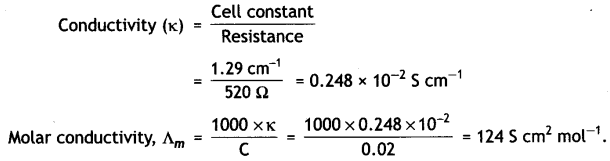OR

(i) Faraday’s first law of electrolysis states that the amount of any substance deposited or liberated at any electrode is directly proportional to the quantity of electricity passed through the electrolytic solution.
Cu2+ + 2e → Cu(s)
Reduction of 1 mol of Cu2+ ion requires 2F of electricity.

(ii) The electrode reactions and cell reaction are
Mg(s) → Mg2+(aq) + 2e (at anode)
Cu2+(aq) + 2e → Cu(s) at cathode
Mg(s) + Cu2+(aq) → Mg2+(aq) + Cu(s)= 2.71 – 0.0295
= 2.6805 v.

Question 16.
(i) How many moles of mercury will be produced by electrolysing 1.0 M Hg(NO3)2 solution with a current of 2.00 A for 3 hours? [Hg(NO3)2 = 200.6 g mol-1].
Hg2+ + 2e → Hg
Quantity of electricity passed = 1 × t(sec)
= 2.0 A × 3.0 × 60 × 60 = 21 600C
2 × 96500 C of electricity produces mercury = 1 mol
21,600 C of electricity will produce mercury
= $$\frac{1}{2 \times 96500}$$ × 21600 = 0.112 mol

(ii) A voltaic cell is set up at 25° C with the following half cells:
Al3+ (0.001 M) and Ni2+ (0.50 M).
Write an equation for the reaction that occurs when the cell generates an electric current and determine the cell potential.
(Given: E°Ni2+/Ni = – 0.25 V, E°Ni2+/Ni = – 1.66V) (CBSE 2011)
Cell reaction is: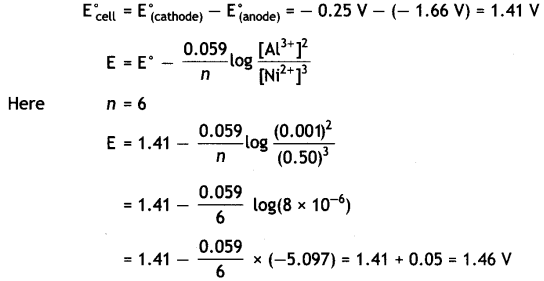Question 17.
(i) What type of battery is lead storage battery? Write the anode and cathode reactions and the overall cell reaction occurring in the operation of a lead storage battery.
It is secondary cell.
Anode reaction:
Pb (s) + SO42-(aq) → PbSO4(s) + 2e

Cathode reaction:
PbO2 (s) + 4H+(aq) + SO42-(aq) + 2e → PbSO4(s) + 2H2O (l)

Overall reaction:
Pb (s) + PbO2 (s) + 2H2SO4 → PbSO4(s) + 2H2O (l)

(ii) Calculate the potential for half cell containing 0.1 M K2Cr2O7(aq), 0.20 M Cr3+(aq) and 1.0 × 10-4 M H+(aq). The half cell reaction is
Cr2O72-(aq) + 14 H+(aq) + 6e → 2Cr3+(aq) + 7H2O(l),
and the standard electrode potential is given as E° = 1.33 V. (CBSE 2011)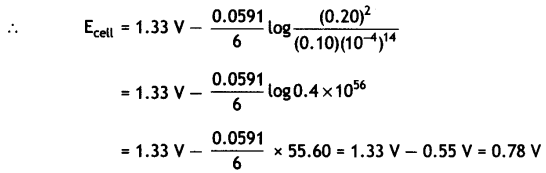Question  18.
(i) State Kohlrausch law of independent migration of ions. Write an expression for the molar conductivity of acetic acid at infinite dilution according to Kohlrausch law.
Kohlrausch law states that at infinite dilution, each ion makes a definite contribution towards molar conductivity of the electrolyte irrespective of the nature of the other ion with which it is associated. This means that the molar conductivity at infinite dilution for a given salt can be expressed as the sum of the individual contributions from the ions of the electrolyte.

For acetic acid, limiting molar conductvity at infinite dilution can be written as:
∧°(CH3COOH) = λ° (CH3COO) + λ° (H+) ……. (i)
This equation can be obtained by the knowledge of molar conductivity at infinite dilution for some electrolytes. For example, consider the strong electrolytes HCl, NaCl and CH3COONa. From Kohlrausch’s law:
∧°(CH3COONa) = λ°(CH3COO) + A°(Na+) …… (i)
∧°(HCl) = λ°(H+) + λ°(Cl) …….. (ii)
∧° (NaCl) = λ° (Na+) + λ° (Cl) …….. (iii)
It is clear that
λ° (CH3COO) + λ° (H+) = [λ°(CH3COO) + A°(Na+)] + [λ°(H+) + λ°(Cl)] – λ° (Na+) + λ° (Cl)
∧°(CH3COOH) = ∧°(CH3COONa) + ∧°(HCl) – ∧° (NaCl)

(ii) Calculate ∧°m for acetic acid
Given that ∧°m(HCl) = 426 S cm2 mol-1
∧°m(NaCl) = 126 S cm2 mol-1
∧°m(CH3COONa) = 91 S cm2 mol-1 (CBSE Delhi 2010)
We know
∧°(CH3COOH) = ∧°(CH3COONa) + ∧°(HCl) – ∧° (NaCl)
= 91 + 426 – 126 = 391 S cm2 mol-1

Question 19.
cell for the given redox reaction is 2.71 V.
Mg(s) + Cu2+(0.01 M) → Mg2+(0.001 M) + Cu(s)
Calculate Ecell for the reaction. Write the direction of flow of current when an external opposite potential applied is
(i) less than 2.71 V and
(ii) greater than 2.71 V
Mg(s) + Cu2+(0.01 M) → Mg2+(0.001M) + Cu(s)= 2.71 – 0.0295 log 10-1
= 2.71 + 0.0295
= 2.7395 V or approximately 2.74 V
(i) When Eext < 2.71, current flows from Cu to Mg
(ii) When Eext > 2.71, current flows from Mg to Cu.

OR

(a) A steady current of 2 amperes was passed through two electrolytic cells X and Y connected in series containing electrolytes FeS04 and ZnS04 until 2.8 g of Fe deposited at the cathode of cell X. How long did the current flow? Calculate the mass of Zn deposited at the cathode of cell Y. (Molar mass: Fe = 56 g mol-1, Zn = 65.3 g mol-1, 1F = 96500 C mol-1)
(b) In the plot of molar conductlvtty (∧m) vs square root of concentration (c1/2), the following curves are obtained for two electrolytes A and B: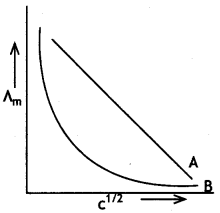(i) Predict the nature of electrolytes A and B.
(ii) What happens on extrapolation 0f ∧m to concentration approaching zero for electrolytes A and B? (CBSE Delhi 2019)
(a) Cell-X contains FeSO4
FeSO4(aq) → Fe2+ + SO42-
Fe2+ + 2e → Fe (at cathode)
1 mole or 56g of Fe is deposited by 2 × 96500C
2.8g of Fe with be deposited by= 2 × 96500 × 2.8
= 9650 C
Q = I × t
9650 = 2 × t
or t = $$\frac{9650}{2}$$ = 4825s
In cell Y
1 mol or 65.3 g of Zn is deposited by 2 × 96500 C
or 2 × 96500 C of electricity will deposit Zn = 65.3 g
9650 C of electricity will deposit Zn = $$\frac{65.3 \times 9650}{2 \times 96500}$$ = 3.265 g

(b) (i) Electrolyte A is strong electrolyte.
(ii) Electrolyte B is weak electrolyte.
(iii) For A, the intercept will give ∧°m.
For B, we cannot obtain ∧°m on extrapolation.

Question 20.
(i) Define molar conductivity of a solution and explain how molar conductivity changes with change in concentration of solution for a weak and a strong electrolyte.
Molar conductivity is the conducting power of all the ions produced by dissolving one gram mole of an electrolyte in solution. It is expressed as ∧m and is defined as:
m = $$\frac{\kappa \times 1000}{M}$$
where M is the molarity of the solution. Its units are ohm-1 cm2 mol-1.
The molar conductance of an electrolytic solution decreases with increase in concentration. For weak electrolytes, molar conductivity increases sharply with dilution. On the other hand, for strong electrolytes, molar conductivity increases slowly with dilution.

For weak electrolytes, the increase in molar conductivity is due to increase in degree of ionisation with dilution. For strong electrolytes, the increase in molar conductance with dilution is because of decrease in interactions between ions with dilution. The decrease in molar conductivity for weak electrolyte (CH3COOH) and strong electrolyte (KCl) is shown below:(ii) The resistance of a conductivity cell containing 0.001 M KCl solution at 298 K is 1500 Ω. What is the cell constant if the conductivity of 0.001 M KCl solution at 298 K is 0.146 × 10-3 S cm-1 ? (CBSE Delhi 2012)
R = 1500 Ω
Molarity = 0.001 M
K = 0.146 × 10-3S cm-1
$$\frac{1}{A}$$ = ?
Conductivity (κ) = Conductance (G) × Cell Constant $$\left(\frac{1}{A}\right)$$
or κ = $$\frac{1}{R} \times \frac{l}{A}$$
or Cell constant $$\frac{1}{A}$$ = R × κ
= 1500 Ω × 0.146 × 10-3S cm-1
$$\frac{1}{A}$$ = 0.219 cm-1

Question 21.
(i) What type of a battery is the lead storage battery ? Write the anode and the cathode reactions and the overall reaction occurring in a lead storage battery when current is drawn from it.
It is secondary cell.
Anode reaction:
Pb (s) + SO42-(aq) → PbSO4(s) + 2e

Cathode reaction:
PbO2 (s) + 4H+(aq) + SO42-(aq) + 2e → PbSO4(s) + 2H2O (l)

Overall reaction:
Pb (s) + PbO2 (s) + 2H2SO4 → PbSO4(s) + 2H2O (l)

(ii) In the button cell, widely used in watches, the following reaction takes place
Zn(s) + Ag2O(s) + H2O(l) → Zn2+(aq) + 2Ag(s) + 2OH(aq)
Determine E° and ∆G° for the reaction.
(given: E°Ag2+/Ag = + 0.80V, E°Zn2+/Zn = – 0.76V)